Maths-
General
Easy

Question

# The lengths of the two sides of right triangle containing the right angle differ by 2 cm. If the area of triangle is 24 cm2. Find the perimeter of triangleHint:

## The correct answer is: 24 cm

### It is given that difference between perpendicular and base = 2 cmi.e. b – h = 2  ⇒  b = 2 + hNow, Area of triangle = 24 cm2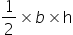= 24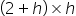= 48h2 + 2h – 48 = 0h =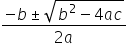h =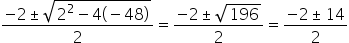h = 6 , - 8Since, perpendicular is always positive so h = 6Base, B = 2 + 6 = 8Using Pythagoras theorem ,H2 = B2 + P2H2 = 82 + 62 = 64 + 36 = 100H =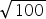= 10 cmPerimeter of triangle = Sum of all sides= 8 + 10 + 6 = 24 cm#### With Turito Foundation.#### Get an Expert Advice From Turito.